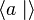# Presentation of free product is disjoint union of presentations

Jump to: navigation, search

## Statement

### For two groups

Suppose$G_1$ and$G_2$ are groups given by presentations. Then, a presentation for the external free product$G_1 * G_2$ is given as follows: we first make sure that all the letters for generators in the presentation of$G_1$ are different from the letters in the presentation of$G_2$ (i.e., we make disjoint the sets of generators). Now, we take the generating set for$G_1 * G_2$ as the union of both these generating sets and the relation set for$G_1 * G_2$ as the union of the relations for$G_1$ and$G_2$.

### For a collection of more than two groups

The same rule applies: we make pairwise disjoint generating sets for all the groups, then take a disjoint union of the generators and of the relations.

## Examples

In the examples, we use$1$ to stand for the identity element. To avoid confusion, we already make disjoint the presentations for the two groups for which we are taking the free product.$G_1$ Presentation$G_2$ Presentation$G_1 * G_2$ Presentation
group of integers$\langle a \mid \rangle$ group of integers$\langle b \mid \rangle$ free group:F2$\langle a,b \mid \rangle$
cyclic group:Z2$\langle a \mid a^2 = 1 \rangle$ group of integers$\langle b \mid \rangle$  ?$\langle a,b \mid a^2 = 1 \rangle$
cyclic group:Z2$\langle a \mid a^2 = 1 \rangle$ cyclic group:Z2$\langle b \mid b^2 = 1 \rangle$ infinite dihedral group$\langle a,b \mid a^2 = b^2 = 1 \rangle$

More examples should be added to illustrate free product with more complicated presentations